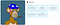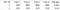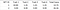# Ranking Rarity: Understanding Rarity Calculation Methods

As noted in the Introducing rarity.tools article, one of the most common questions people ask in the discords of collectible NFT projects is ‘how rare is my nft’

This is because rarity is one of the most important factors in determining the value of an individual NFT.

But how do you determine the overall rarity of an individual NFT piece? That is what this series of articles is going to (try to) answer.

# The Problem

If you look at any collectible NFT item on OpenSea, such as a Bored Ape Yacht Club, you will see that it has many properties (or traits).

From looking at the properties, you may be able to determine that an NFT has some rare traits.

But how rare is this NFT compared to others? When comparing two NFTs do you simply compare the rarest trait of each NFT?Bored Ape Yacht Club #9941’s Properties .. is he rarer than #73 above?

Because each NFT has multiple traits, there has to be a way to combine the rarity of all the traits into one single value per NFT to be able to actually rank them.

There have been many ways people have been ranking NFTs by rarity. Below we talk about some of them.

# Trait Rarity Ranking

This refers to comparing NFTs by simply comparing the rarest trait of each NFT. For example, comparing the Apes above:

• Ape #73 s rarest trait is Sold Gold Fur which 0.46% have
• Ape #9941's rarest trait is Bored Unshaven Dagger which 0.28% have
• Ape #9542’s rarest trait is Bored Unshaven Pizza which 0.26% have

Using ‘Trait Rarity Ranking’ then the order would be #9542, #9941 and last #73

While it is a simple straightforward method, the weakness of this method is it only considers the rarest trait of each NFT.

Imagine we had a collection of NFTs that have 4 traits each. Suppose we had 2 NFTs that we wanted to compare as in the table below:

Using Trait Rarity, NFT ID 1 would win because it’s rarest trait (Trait 1) has 10% rarity which is less than any of NFT 2’s traits. But the rest of NFT 2’s traits are all a lot rarer than all the rest of NFT 1’s traits. Overall wouldn’t NFT 2 be valued more?

And that is the weakness of ranking by Trait Rarity. It doesn’t look at the overall rarity of the NFTs at all, just the rarest trait.

# Average Trait Rarity

Another method that is sometimes used is averaging the rarity of traits that exist on the NFT.

For example if an NFT had 2 traits, one with 50% rarity and another with 10% rarity, it’s average trait rarity would be (50+10)/2 = 30%

For our apes:

• Ape #73’s average trait rarity is 4.05%
• Ape #9941’s average trait rarity is 6.056%
• Ape #9542’s average trait rarity is 6.452%

So with this method, the order would be completely flipped from the previous method. The ranking would be #73, #9941 and then #9542

Is Average Trait Rarity any good? Well, at least it considers the overall rarity of the traits. Let’s look at that previous example with NFT ID 1 and 2 againExample Collection A: Average trait rarity is calculated and listed at the rightmost column.

So the Average rarity of NFT ID 2 is 0.11, while NFT ID 1 is 0.625. That means Average Rarity says NFT ID 2 is rarer than NFT ID 1.

The problem with this method (which Statistical Rarity that is described next also has) is that it puts so much weight on the overall rarity of every trait, that NFTs that have a single super rare trait are not valued enough, as their rarity value gets too ‘diluted’ by the other traits.

To illustrate this, imagine we had a collection of NFTs that looked like this:

Which one would you say is the rarest? Of course it’s NFT ID 1!

Now let’s try using Traity Rarity ranking and Average Trait Rarity ranking on them. First we convert the trait values to their trait rarity percentages:

If we used Trait Rarity ranking on this collection, then NFT ID 1 would be the rarest, which aligns with what we think should be right.

Now lets try using Average Trait Rarity

Oh! Turns out Average Trait Rarity ranking thinks NFT IDs 7, 8, 9 and 10 are rarer than NFT ID 1!

But NFT ID 1 is the only unique one, the only 1 of 1 and obviously would be most valuable in the collection, wouldn’t it?

That means maybe Average Rarity ranking isn’t a really good method after all?

# Statistical Rarity

Now lets look at Statistical Rarity which has become a somewhat popular method and is used very often in community made spreadsheets.

In Statistical Rarity, which as far as I know was first written about in relation to NFTs by Adam Chekroud, you calculate the overall rarity of an NFT by multiplying all of it’s trait rarities together.

For example if an NFT has 2 traits with 1 trait at 10% and the other trait at 50%, the ‘statistical rarity’ for that NFT would be (10% * 50%) = 5%

For our 3 apes, in order to make the result make sense, we need to add that there is a 22.56% chance for apes to have no hat, 70.23% chance for apes to have no earrings and 18.8% chance for apes to have no clothes. (It is arguable that these should have been added in the ‘Average Trait Rarity’ calculation too but it would not have changed the end result, instead it would have only made it more extreme)

• Ape #73’s statistical rarity is 0.00000000070744%
• Ape #9941’s statistical rarity is 0.00000056965722%
• Ape #9542’s statistical rarity is 0.00000044983967%

So, with this method, the ranking would be #73, #9542 and then #9941

Let’s recap those rankings:

Three different methods with three different results when comparing just 3 apes. Imagine the differences between them when sorting the full collection of 10,000!

All these methods are methods that are currently being used in community made ranking spreadsheets and websites.

So if this article doesn’t convince you of anything, it should at least convince you that ranking the rarity of NFTs is a problem without an obvious solution.

# Statistical Rarity vs. Example Collection A and B

Now let’s see how Statistical Rarity does with our 2 example collections that we tested Trait Rarity and Average Trait Rarity with.

First let’s see the one Trait Rarity failed with:

Here it says NFT ID 2 is rarer which matches Average Trait Rarity, and so it passes our first test.

Next lets see how it does with Example Collection B

Oh! Just like with Average Trait Rarity, Statistical Rarity says NFT ID 7–10 are rarer than NFT ID 1!

But IDs 7–10 are duplicates of each other! Or should we believe in Statistical Rarity results and go out and buy IDs 7–10 at a higher price than NFT ID 1?

But clearly NFT ID 1 is the only 1 of 1, the rarest if you ask most people as there isn’t any others like it.

Some might say ‘but I thought Statistical Rarity was ‘Statistically Correct’ ’?

The thing is, by multiplying all the rarities of each NFT’s traits, you aren’t really measuring the rarity of an NFT in a specified NFT collection. What is really being measured is something else, which I will leave for the reader to ponder as this article would get even longer than it is.

Lets look at Rarity Score next.

# Rarity Score: How is it Calculated?

So, what is Rarity Score? Rarity Score is a method that I (crazy4nfts) came up with when I created WFBrowser, the original ranking site whose codebase I built upon to create rarity.tools

The simple way to calculate Rarity Score which was also described on that site is:

[Rarity Score for a Trait Value] = 1 / ([Number of Items with that Trait Value] / [Total Number of Items in Collection])

The total Rarity Score for an NFT is the sum of the Rarity Score of all of it’s trait values.

This simple calculation gives very good results and today is also used as the basis of rarity ranking in other NFT sites including an NFT marketplace.

Because [Number of Items with that Trait Value] / [Total Number of Items in Collection] is the same as the trait rarity (fractional, not %), we can also say that

[Rarity Score for a Trait Value] = 1 / [Trait Rarity of that Trait Value]

# Rarity Score vs. Example Collection A and B

Let’s go ahead and see how Rarity Score tackles our two problem examples.

Remember that these imaginary NFT collections are extremely simple. If whatever calculation method we use can’t even get these right, then how can we trust our method to get collections of 10,000s of NFTs right?

Let’s start with Example Collection A. First we write down the trait rarities in fractional form:

Then we convert them to rarity scores. For example NFT ID 1 Trait 1 Rarity Score is (1 / 0.1) = 10 and then we add them all up for each NFT IDExample Collection A: Rarity Score for each Trait Value and Total Rarity Score for each NFT ID

So Rarity Score says NFT ID 2 is more valuable because it has a higher score!

Next let’s see how it handles Example Collection B. We’ll go ahead and calculate the scores:

Yes! Rarity Score correctly ranks NFT ID 1 as the most rare NFT followed by NFT IDs 7–10 and then IDs 2–6.

So what we have seen here is in both of these example cases, the Rarity Score method has given us results that match our instinctive human picks of which NFT is rarer, while Statistical Rarity and other methods have failed.

# Summary

As noted, Statistical Rarity and Average Rarity have a tendency to over-emphasize the overall level of rarities of all traits in an NFT, while not giving enough emphasis to single rare traits that could be 1 of 1s in the whole collection.

Trait Rarity on the other hand has the complete opposite problem where it only considers the rarest trait.

Rarity Score give results that give enough emphasis to single rare traits and also includes overall trait rarities in its calculation. And most importantly the results it gives match better with our human expectations.

# What’s Next?

While the calculation methods are the most core part of calculating the rankings, there are still many additional elements that are used to get the best final result.

In the next articles, I will write about trait normalization, uniqueness, weightings and using combined traits and possibly other topics.

Why or how Rarity Score works is also something I might write about.

https://rarity.tools : NFTs ranked and sorted by rarity! See the rarest CryptoPunks, Hashmasks, Waifusions and Chubbies and check how rare a specific ID is.

## More from rarity.tools

https://rarity.tools : NFTs ranked and sorted by rarity! See the rarest CryptoPunks, Hashmasks, Waifusions and Chubbies and check how rare a specific ID is.